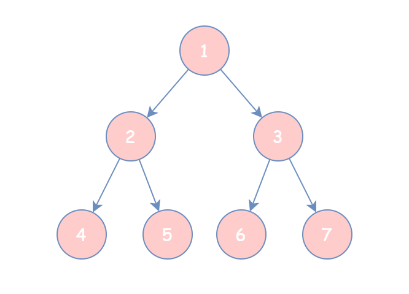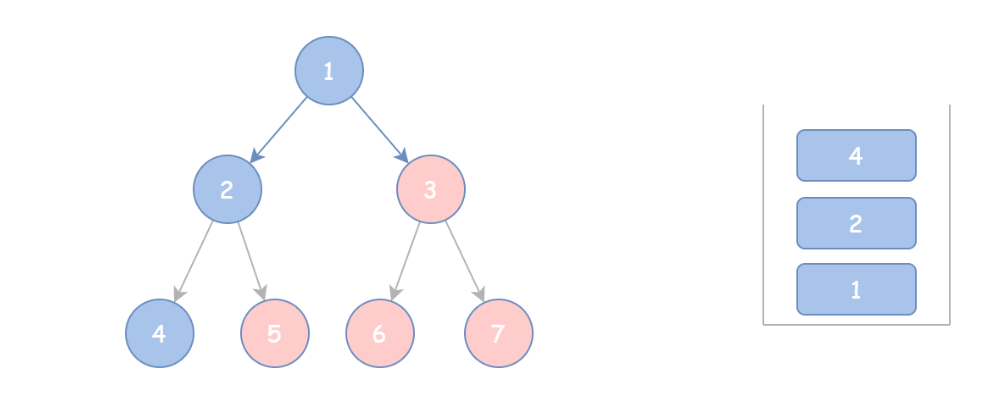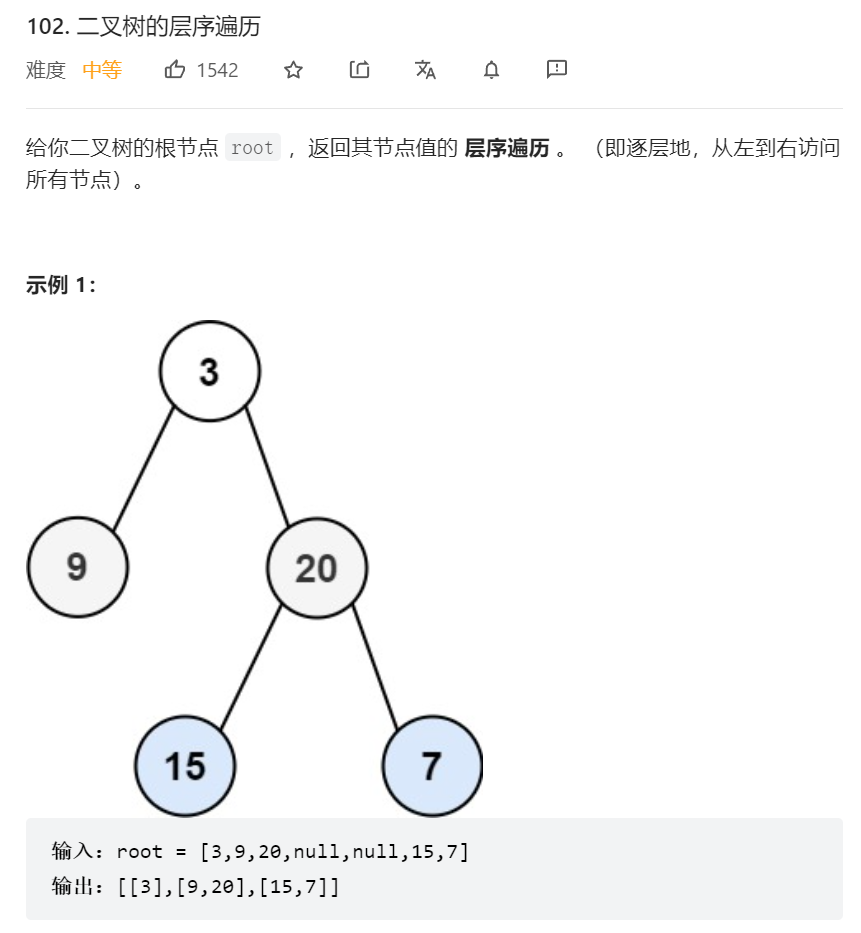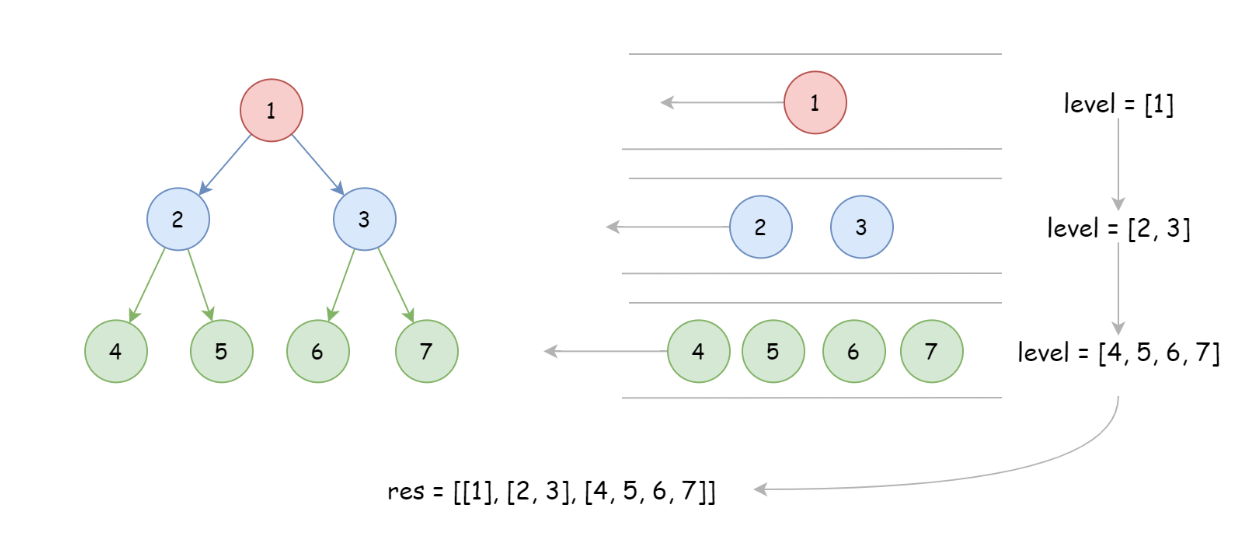## [Leetcode] 二叉树的遍历

news/2023/12/8 21:27:49

# 1. 相关题目

144.二叉树的前序遍历 https://leetcode.cn/problems/binary-tree-preorder-traversal/

94. 二叉树的中序遍历 https://leetcode.cn/problems/binary-tree-inorder-traversal

145. 二叉树的后序遍历 https://leetcode.cn/problems/binary-tree-postorder-traversal/submissions/

102. 二叉树的层序遍历 https://leetcode.cn/problems/binary-tree-level-order-traversal/

# 2. 基本概念

## 遍历方式# 3. 题目解析

## 3.1 递归解法

``def dfs(root):if not root:returnres.append(root.val)dfs(root.left)dfs(root.right)``

### 3.1.1 前序遍历

``````# Definition for a binary tree node.
# class TreeNode:
#     def __init__(self, val=0, left=None, right=None):
#         self.val = val
#         self.left = left
#         self.right = right
class Solution:def inorderTraversal(self, root: Optional[TreeNode]) -> List[int]:res = []def dfs(root):if not root:returndfs(root.left)res.append(root.val)dfs(root.right)dfs(root)return res``````

### 3.1.2 中序遍历

``class Solution:def preorderTraversal(self, root: Optional[TreeNode]) -> List[int]:res = []def dfs(root):if not root:returndfs(root.left)res.append(root.val)dfs(root.right)dfs(root)return res``

### 3.1.3 后序遍历

``class Solution:def postorderTraversal(self, root: Optional[TreeNode]) -> List[int]:res = []def dfs(root):if not root:returndfs(root.left)dfs(root.right)res.append(root.val)dfs(root)return res``

## 3.2 迭代解法

### 3.2.1 前序遍历

#### 3.2.1.1 层层入栈

``class Solution:def preorderTraversal(self, root: Optional[TreeNode]) -> List[int]:stack, res = [root], []while stack:node = stack.pop()if node:#根节点的值加入到结果中res.append(node.val) #右子树入栈if node.right:stack.append(node.right)#左子树入栈if node.left:stack.append(node.left) return res                    ``

#### 3.2.1.3 层层入栈（使用标志位）

``class Solution:def preorderTraversal(self, root: Optional[TreeNode]) -> List[int]:if not root:return []stack, res = [(0,root)], []while stack:flag, node = stack.pop()#if not node: continueif node:#遍历过了，加入结果if flag == 1:res.append(node.val)else:stack.append((0,node.right)) #右stack.append((0,node.left)) #左stack.append((1,node)) #根return res``

#### 3.2.1.2 根节点和左孩子先入栈``class Solution:def preorderTraversal(self, root: Optional[TreeNode]) -> List[int]:if not root:return []cur, stack, res = root, [], []while cur or stack:while cur:#根节点和左孩子入栈res.append(cur.val)stack.append(cur)cur = cur.left#每弹出一个元素就达到右孩子tmp = stack.pop()cur = tmp.rightreturn res ``

### 3.2.2 中序遍历

#### 3.2.2.1 层层入栈（使用标志位）

``class Solution:def inorderTraversal(self, root: Optional[TreeNode]) -> List[int]:if not root:return []stack, res = [(0,root)], []while stack:flag, node = stack.pop()#if not node: continueif node:#遍历过了，加入结果if flag == 1:res.append(node.val)else:stack.append((0,node.right)) #右stack.append((1,node)) #根stack.append((0,node.left)) #左return res``

#### 3.2.2.2 根节点和左孩子先入栈

``class Solution:def inorderTraversal(self, root: Optional[TreeNode]) -> List[int]:if not root:return []cur, stack, res = root, [], []while cur or stack:while cur:#根节点和左孩子入栈,到达最左端的叶子节点stack.append(cur)cur = cur.lefttmp = stack.pop()#出栈时再加入结果res.append(tmp.val)cur = tmp.right ``

### 3.2.3 后序遍历

#### 3.2.3.1 层层入栈（使用标志位）

``class Solution:def postorderTraversal(self, root: Optional[TreeNode]) -> List[int]:if not root:return []stack, res = [(0,root)], []while stack:flag, node = stack.pop()#if not node: continueif node:#遍历过了，加入结果if flag == 1:res.append(node.val)else:stack.append((1,node)) #根stack.append((0,node.right)) #右stack.append((0,node.left)) #左return res``

#### 3.2.3.2 根节点和左孩子先入栈

1.参考前序遍历的实现，可以达到输出 “根 -> 右 -> 左 ”，逆序即为预期输出。

2.后序遍历采用模板解法并没有按照真实的栈操作，而是利用了结果的特点反向输出。

``class Solution:def postorderTraversal(self, root: Optional[TreeNode]) -> List[int]:if not root:return []cur, stack, res = root, [], []while cur or stack:while cur:#先到达最右端res.append(cur.val)stack.append(cur)cur = cur.righttmp = stack.pop()cur = tmp.leftreturn res[::-1]``

## 3.3 层序遍历``class Solution:def levelOrder(self, root: Optional[TreeNode]) -> List[List[int]]:if not root:return []res, q = [], [root]while q:n = len(q)level = []for i in range(n):#从队列中逐个弹出该层的每个节点node = q.pop(0)level.append(node.val)#将节点的左右孩子加到队尾，供下一层遍历使用q.append(node.left) if node.left else 1q.append(node.right) if node.right else 1res.append(level)return res``

### RabbitMQ通配符模式

&#x1f341;博客主页&#xff1a;&#x1f449;不会压弯的小飞侠 ✨欢迎关注&#xff1a;&#x1f449;点赞&#x1f44d;收藏⭐留言✒ ✨系列专栏&#xff1a;&#x1f449;Linux专栏 &#x1f525;欢迎大佬指正&#xff0c;一起学习&#xff01;一起加油&#xff01; 目录&…

### 【云原生进阶之容器】第二章Controller Manager原理2.5节--DeltaFIFO剖析

5 DeltaFIFO DeltaFIFO是K8s中用来存储处理数据的Queue，相较于传统的FIFO，它不仅仅存储了数据保证了先进先出，而且存储有K8s 资源对象的类型，它的作用是保证Reflector和Indexer之间对象同步。其是连接Reflector(生产者)和indexer(消费者)的重要通道。其核心处理流程如下： …

### 驱动之设备模型

1. 起源与新方案 1.1 起源 仅devfs&#xff0c;导致开发不方便以及一些功能难以支持 热插拔不支持一些针对所有设备的同意操作&#xff08;如电源管理&#xff09;不能自动mknod用户查看不了设备信息设备信息硬编码&#xff0c;导致驱动代码通用性差&#xff0c;即没有分离设…

### C++——命名空间，输入输出，缺省参数

✅<1>主页&#xff1a;我的代码爱吃辣 &#x1f4c3;<2>知识讲解&#xff1a;数据结构——二叉树 &#x1f525;<3>创作者&#xff1a;我的代码爱吃辣 ☂️<4>开发环境&#xff1a;Visual Studio 2022 &#x1f4ac;<5>前言&#xff1a;补充C语言…

### 通信原理 | 基本概念

1 通信及通信系统 通信（Communication）是实现信息和消息传输的过程 通信系统（Communication System）的组成： 实现通信的所有硬件和软件设备、传输媒介以及各种通信协议等 消息（Message）、信息（Information）、信号（Signal）的区别： 消息通常指人的感官能够感受到的…